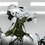# Programming a Conditional Probability Problem

Hi all. I have been working my way through the Probability course, specifically the section on Conditional Probability, when I fell upon this linked problem.

By solving the linked problem recursively, you find that you have a $\frac{1}{3}$ probability of winning the game.

I thought this would be a fun problem to program, so I wrote a program in Python 3 to solve this using Monte Carlo methods. The program and output of the program can be seen below.

  1 2 3 4 5 6 7 8 9 10 11 12 13 14 15 16 17 18 19 20 21 from random import choice trials = 10000000 #number of times the game is played (10,000,000) lose = 0 win = 0 for game in range(1, trials): options = [1,2,3,4,5,6] #can roll any of these numbers chips = 1 #starting point for each game while chips > 0 and chips < 4: #continue this loop for as long as you haven't lost or won roll = choice(options) #picking random number out of options, like rolling a die if roll == 1 or roll == 2 or roll == 3: chips -= 1 elif roll == 4 or roll == 5: chips += 1 elif roll == 6: chips += 2 if chips <= 0: lose += 1 elif chips >= 4: win += 1 print(win/lose) #probability of winning 

Output:

0.5001471644515492


Assuming the program is correct, the probability of winning the game is approximately $\frac{1}{2}$, as opposed to the original solution that states you have a $\frac{1}{3}$ chance of winning the game.

Can anyone explain what is going on here (it is very possible that my code has something wrong with it, or I am misunderstanding some fundamental concept. If you catch anything please point it out :)?Note by Sam Everett
3 years, 2 months ago

This discussion board is a place to discuss our Daily Challenges and the math and science related to those challenges. Explanations are more than just a solution — they should explain the steps and thinking strategies that you used to obtain the solution. Comments should further the discussion of math and science.

When posting on Brilliant:

• Use the emojis to react to an explanation, whether you're congratulating a job well done , or just really confused .
• Ask specific questions about the challenge or the steps in somebody's explanation. Well-posed questions can add a lot to the discussion, but posting "I don't understand!" doesn't help anyone.
• Try to contribute something new to the discussion, whether it is an extension, generalization or other idea related to the challenge.

MarkdownAppears as
*italics* or _italics_ italics
**bold** or __bold__ bold
- bulleted- list
• bulleted
• list
1. numbered2. list
1. numbered
2. list
Note: you must add a full line of space before and after lists for them to show up correctly
paragraph 1paragraph 2

paragraph 1

paragraph 2

[example link](https://brilliant.org)example link
> This is a quote
This is a quote
    # I indented these lines
# 4 spaces, and now they show
# up as a code block.

print "hello world"
# I indented these lines
# 4 spaces, and now they show
# up as a code block.

print "hello world"
MathAppears as
Remember to wrap math in $$ ... $$ or $ ... $ to ensure proper formatting.
2 \times 3 $2 \times 3$
2^{34} $2^{34}$
a_{i-1} $a_{i-1}$
\frac{2}{3} $\frac{2}{3}$
\sqrt{2} $\sqrt{2}$
\sum_{i=1}^3 $\sum_{i=1}^3$
\sin \theta $\sin \theta$
\boxed{123} $\boxed{123}$

Sort by:

Excellent simulation. Your code is mostly correct, except for a small typo in line 21. Can you figure it out?

- 3 years, 2 months ago